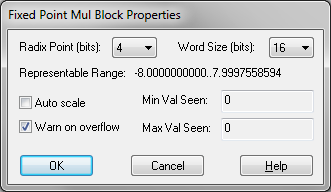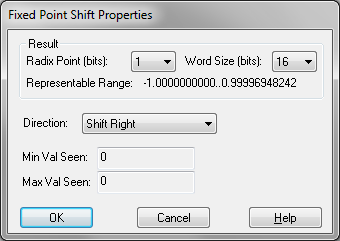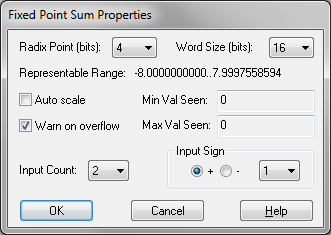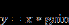## 1/XBlock Category: Arithmetic

Inputs: Real or complex scalars, vectors, or matrices.

Description: The 1/X block generates the inverse of the input signal. If a vector or matrix is fed into a 1/X block, the result is an element-by-element inversion of the vector or matrix (that is, [one divided by the element] operation). This is not equivalent to a normal vector pseudo-inverse operation or a normal matrix inverse operation.

Use the invert block to compute the matrix inverse of a matrix.Label: Indicates a user-defined block label that appears when View > Block Labels is activated.

#### Examples

1. Computation of 1/X of a scalar

Consider the equation y= 1/25 realized as:The incoming constant value of 25 results in 1/25 = 0.04.

2. Computation of 1/X of a vector

Consider the equation:

z = 1/y

where y = [-1  5.6  4], and where an element-by-element inversion is implied. This equation is realized as:An element-by-element inverse operation is performed on the three elements in the scalarToVec block.

3. Computation of 1/X of a matrix

Consider the equation:

Z =  1/ Y

whereThis is realized as:When the simulation runs, the 1/X block performs an element-by-element inverse operation on the incoming matrix.